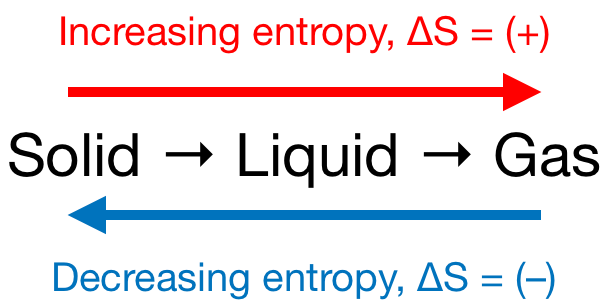# Problem: For which of the following reactions is ∆S° &gt; 0 at 25°C?A) 2H2(g) + O2(g) → 2H2O(g)B) 2ClBr(g) → Cl2(g) + Br2(g)C) I2(g) → I2(s)D) 2NO(g) + O2(g) → 2NO2(g)E) NH4HS(s) → NH3(g) + H2S(g)

###### FREE Expert Solution

We’re being asked to determine the reaction that has ΔS° > 0 at 25°C. Recall that if:

• ΔS > 0 or ΔS = (+); entropy is increased

• ΔS < 0 or ΔS = (–); entropy is decreased

Remember that phase changes lead to a change in entropy:Also, recall that an increase in the number of gas molecules in the reaction also leads to an increase in entropy.

100% (288 ratings)###### Problem Details

For which of the following reactions is ∆S° > 0 at 25°C?

A) 2H2(g) + O2(g) → 2H2O(g)

B) 2ClBr(g) → Cl2(g) + Br2(g)

C) I2(g) → I2(s)

D) 2NO(g) + O2(g) → 2NO2(g)

E) NH4HS(s) → NH3(g) + H2S(g)